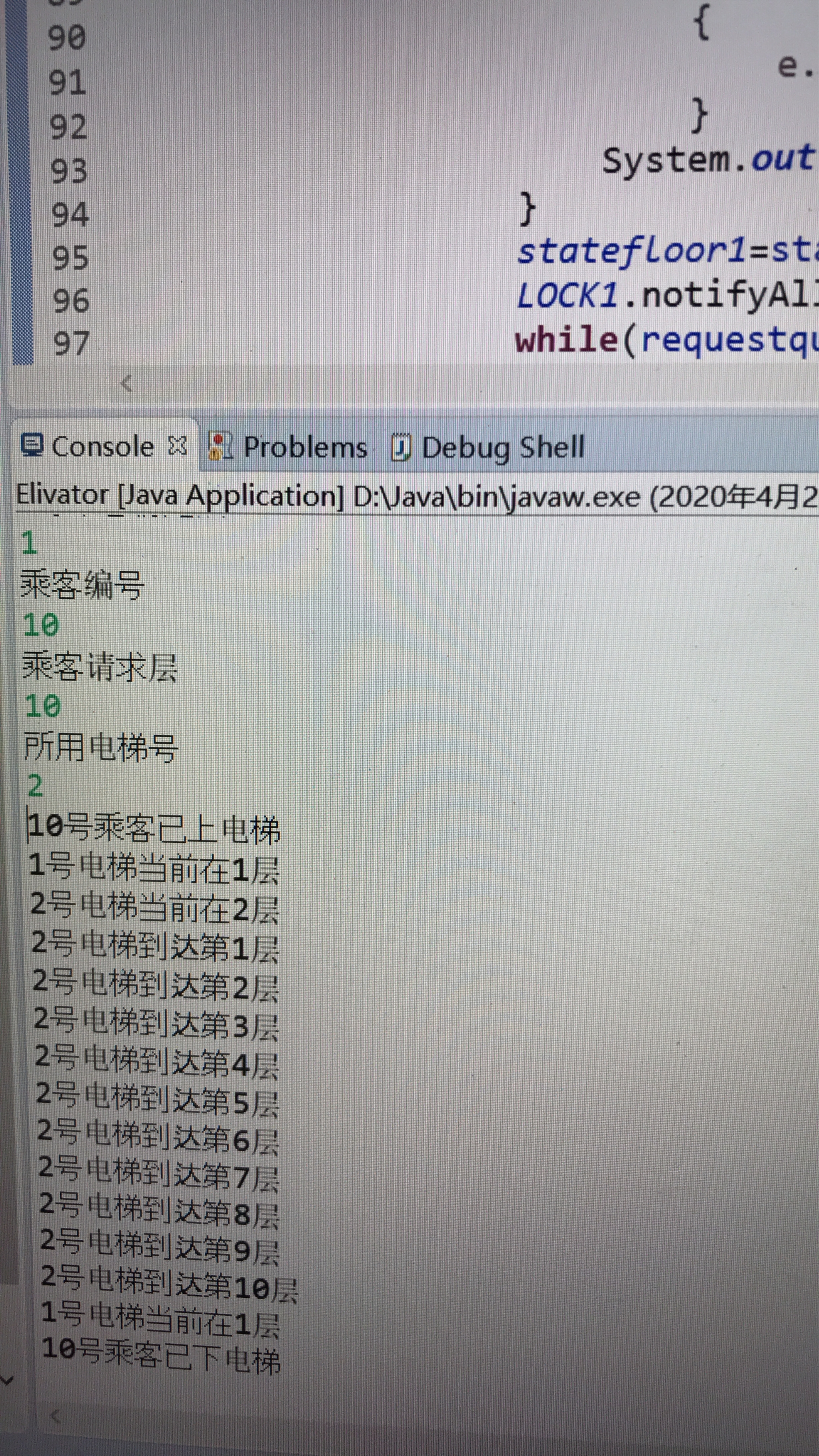# 软件工程第四次作业

    private static String LOCK1="LOCK1";
private static String LOCK2="LOCK2";
static int statefloor1=1;
static int statefloor2=1;
static List<Passenger> requestque1=new ArrayList<Passenger>();
static List<Passenger> requestque2=new ArrayList<Passenger>();
static List<Passenger> requestque3=new ArrayList<Passenger>();
static List<Passenger> requestque4=new ArrayList<Passenger>();

int inifloor;
int name;
int requestfloor;
int statefloor;
int elenumber;
int state;
Passenger()
{
inifloor=1;
statefloor=1;
state=0;
requestfloor=2;
}
Passenger(int inifloor,int requestfloor,int elenumber,int name)
{
this.inifloor=inifloor;
this.requestfloor=requestfloor;
statefloor=inifloor;
this.elenumber=elenumber;
this.name=name;
state=0;
if(elenumber==1)
{
}
if(elenumber==2)
{
}
if(elenumber==3)
{
}
if(elenumber==4)
{
}
}

static class NewElevator1 extends Thread
{
int censhu;
int inifloor;
int statefloor;
int number;
List<Passenger> requestque=new ArrayList<Passenger>();
NewElevator1()
{
inifloor=1;
statefloor=1;
censhu=20;
number=1;
requestque=null;
}
NewElevator1(int censhu,int inifloor,int number)
{
this.censhu=censhu;
this.inifloor=inifloor;
this.number=number;
statefloor=inifloor;
}

***代码链接如下：***

<https://github.com/Trumpetertimes/Trumpetertimes-dock/blob/master/For%204th%20homework%20of%20SE>posted @ 2020-04-29 22:58  Siuhamburger  阅读(208)  评论(0编辑  收藏  举报